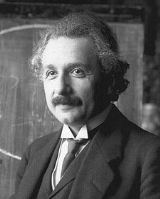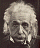xAlbert Einstein
Did Einstein hide on 'Hertz spaces', 'dialectical physics', 'the limit of angular speed'?
Posts  1 - 1  of  1dialecticalphysics

Einstein did not explain on these subjects because he law humanity more than science. He did not say anything on "M.V. Lalan LALAN, M.V. Sur Les Postulats Qui Sont A La Base Des Cinemetiques; Bulletin de la Societe Mathematique de France, Tome 65 (1937)83-99” The limit of linear speed depends on direction. Çizgisel Hız Ereyi yöne bağlıdır” konusunda".
Of course he made some calculations; on:"The sum formula of angular velocities" and reached { E=mc2+Id2, d='the limit of angular speed'}
To find is a gymnasium problem:{"m0 makes a movement like, x= a Sin wt", Can you find the effecting force?} That problem is the death of "black hole concept".
Mass is a function of speed. Does it depend on position and time? That problem is the death of "big bang concept". A gymnasium student could find v= c Sin (H l/c), instead of Hubble law (v=H l).